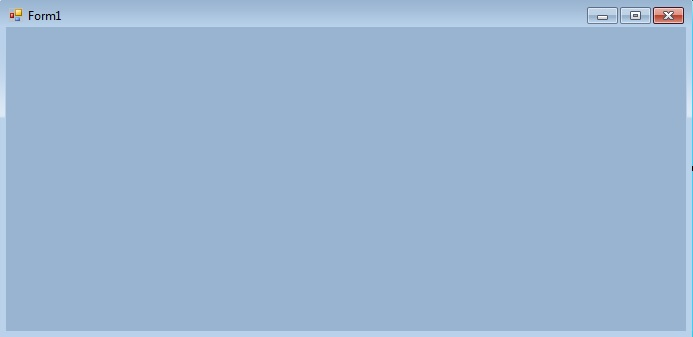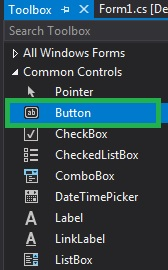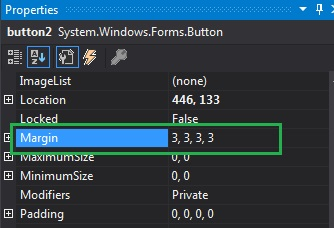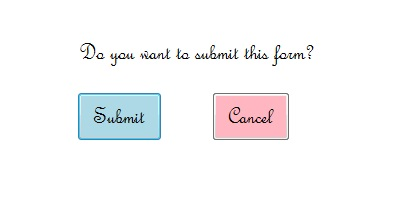Related Articles
How to set the Margin of the Buttons in C#?
• Last Updated : 26 Jun, 2019

A Button is an essential part of an application, or software, or webpage. It allows the user to interact with the application or software. In Button, you are allowed to set the space between two or more Button controls using Margin Property. You can use this property in two different methods:

1. Design-Time: It is the easiest method to set the margin between two button controls. Using the following steps:

• Step 1: Create a windows form as shown in the below image:
Visual Studio -> File -> New -> Project -> WindowsFormApp• Step 2: Drag the Button control from the ToolBox and Drop it on the windows form. You are allowed to place a Button control anywhere on the windows form according to your need.• Step 3: After drag and drop you will go to the properties of the Button control to set the Margin property of the Button.Output:Run-Time: It is a little bit trickier than the above method. In this method, you can set the Margin property of the Button programmatically with the help of given syntax:

`public System.Windows.Forms.Padding Margin { get; set; }`

Here, the Padding is used to represent the space between controls. Following steps are used to set the Margin property of the Button:

• Step 1: Create a button using the Button() constructor is provided by the Button class.
```// Creating Button using Button class
Button MyButton = new Button();
```
• Step 2: After creating Button, set the Margin property of the Button provided by the Button class.
```// Set the margin of the button
Mybutton.Margin = new Padding(5, 5, 5, 5);
```
• Step 3: And last add this button control to form using Add() method.
```// Add this Button to form
```

Example:

 `using` `System; ` `using` `System.Collections.Generic; ` `using` `System.ComponentModel; ` `using` `System.Data; ` `using` `System.Drawing; ` `using` `System.Linq; ` `using` `System.Text; ` `using` `System.Threading.Tasks; ` `using` `System.Windows.Forms; ` ` `  `namespace` `WindowsFormsApp8 { ` ` `  `public` `partial` `class` `Form1 : Form { ` ` `  `    ``public` `Form1() ` `    ``{ ` `        ``InitializeComponent(); ` `    ``} ` ` `  `    ``private` `void` `Form1_Load(``object` `sender, EventArgs e) ` `    ``{ ` ` `  `        ``// Creating and setting the properties of label ` `        ``Label l = ``new` `Label(); ` `        ``l.AutoSize = ``true``; ` `        ``l.Text = ``"Do you want to submit this form?"``; ` `        ``l.Location = ``new` `Point(222, 145); ` `        ``l.Font = ``new` `Font(``"French Script MT"``, 18); ` `        ``// Adding this label to form ` `        ``this``.Controls.Add(l); ` ` `  `        ``// Creating and setting the properties of Button ` `        ``Button Mybutton = ``new` `Button(); ` `        ``Mybutton.Location = ``new` `Point(225, 198); ` `        ``Mybutton.Text = ``"Submit"``; ` `        ``Mybutton.AutoSize = ``true``; ` `        ``Mybutton.BackColor = Color.LightBlue; ` `        ``Mybutton.Padding = ``new` `Padding(6); ` `        ``Mybutton.Font = ``new` `Font(``"French Script MT"``, 18); ` `        ``Mybutton.Margin = ``new` `Padding(5, 5, 5, 5); ` `        ``// Adding this button to form ` `        ``this``.Controls.Add(Mybutton); ` ` `  `        ``// Creating and setting the properties of Button ` `        ``Button Mybutton1 = ``new` `Button(); ` `        ``Mybutton1.Location = ``new` `Point(360, 198); ` `        ``Mybutton1.Text = ``"Cancel"``; ` `        ``Mybutton1.AutoSize = ``true``; ` `        ``Mybutton1.BackColor = Color.LightPink; ` `        ``Mybutton1.Padding = ``new` `Padding(6); ` `        ``Mybutton1.Font = ``new` `Font(``"French Script MT"``, 18); ` `        ``Mybutton1.Margin = ``new` `Padding(5, 5, 5, 5); ` `        ``// Adding this button to form ` `        ``this``.Controls.Add(Mybutton1); ` `    ``} ` `} ` `} `

Output:My Personal Notes arrow_drop_up
Recommended Articles
Page :